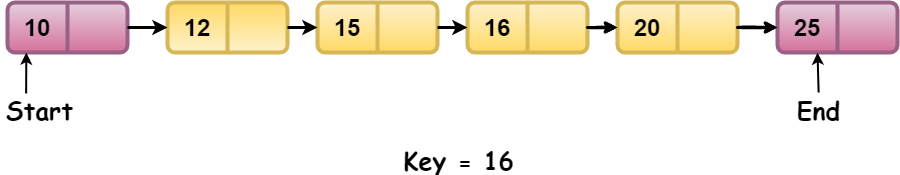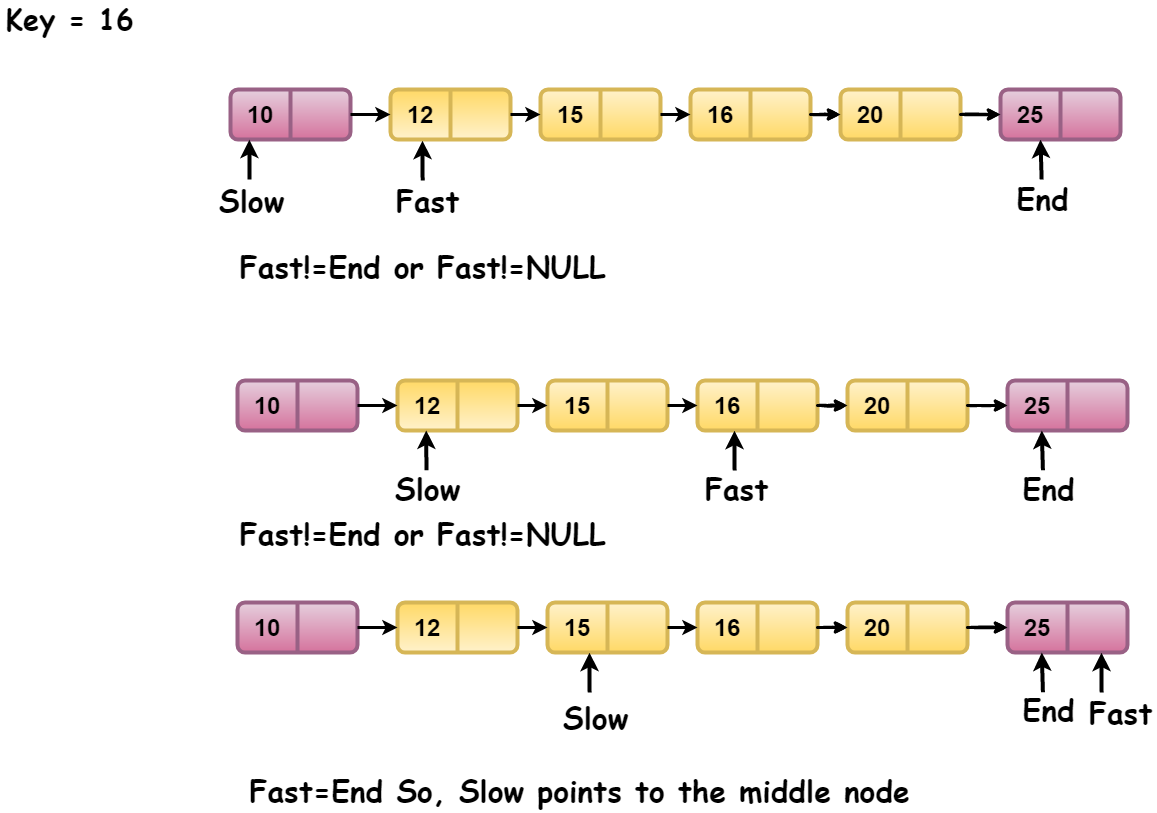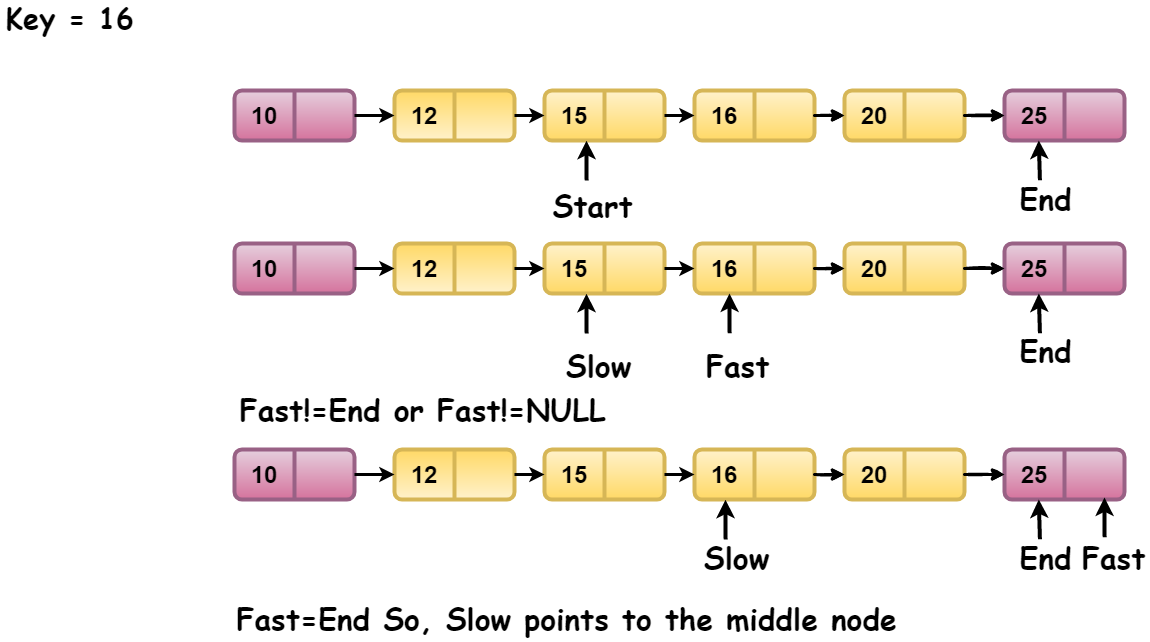# Binary search on Linked List

## Introduction

In this article, we will see how to perform a binary search on a linked list in a simple and precise way.

We know that binary search proves to be useful for searching for a target element when we have a collection of sorted data.

Why?

Because it is not required to iterate over every element, we only search for the target value in a possible range. Being more specific, a divide and conquer approach is adopted, with the search space being halved at each step until we find the target element or the search space is exhausted(element not present).

If you are not aware of binary search, please read Understanding Binary Search first before moving further.

Also, you may check out Introduction to Linked List to brush up quickly.

Let’s get started with the problem statement.

## Problem Statement

You are given a sorted, singly linked list of integers and a target element. You need to find whether the target element is present in the linked list or not.

For example, if the given linked list is-  10->12->15->16->20->NULL and target=12, then since 12 is there in the linked list, so the output is “Target found”. If target=7, then we will print “Target not found”.

For the time being, forget that the linked list is sorted. Just think that we have a linked list and a target element. So, how will you check if the target is there in the list?

Simple. You will traverse the nodes of the linked list and compare the data of each node with the target. If they are equal, the target is present and end the traversal; else, continue until you find the target or reach the end of the list.

This is called Linear Search, and its time complexity is O(N), where is the length of the list.

But in this problem, we are supposed to do a binary search instead of a linear search.

One of the prerequisites to this problem is to find the middle node of a linked list. So, please consider reading the article Finding the Middle Node of a Linked List, as after understanding this concept, the binary search algorithm will be a cakewalk.

## Approach

1. Define two pointers - start and end. The Start points to the head of the linked list and the End points to the last node of the linked list.

2. Find the middle node of the linked list using the fast and slow pointers approach and let it be pointed by the mid pointer.

3. Compare the value of the middle node with the target element. There can be three cases-
a) If mid->value == target:  then print “Target found” and break the loop.

b) If mid->value  < target: then it implies that target lies in the right half of the list as its value is greater than that of the middle node. So, we update the start pointer to point the node next to the middle node, i.e., start=mid->next

c) If mid->value > target: then it means that target lies in the left half of the linked list as its value is lesser than that of the middle node. So, we should search for it in the left half of the list. Thus, we should update the end pointer to point to the node just before the middle node. We have a mid pointer, but we can’t access the node just before it as we don’t have any previous pointer in our node structure. So, we simply update the end pointer as end = mid.

4. Repeat the above steps until the search space is exhausted. That is until the start becomes equal to the end(both pointers pointing to the same node) or the end becomes NULL.

## Dry Run of the Approach

Let’s say we are given a Linked list sorted in ascending order and target/key element.1. Start-> value=10 and End->value=25
Find Middle node-Since, mid->value = 15 and target=16, so target > mid->value. Update Start as Start=mid->next and continue to find the middle node again.

1. Start->value=15 and End->value=25
Let’s find the middle Node-Here, mid->value=16 and target = 16. Since, target = mid->value, so this means we have found the target element in the linked list. So, print “Target element found” and the process is terminated.

Let’s see the code implementation along with the analysis of time and space complexity in the next section.

## C++ Implementation

``````/*C++ code to perform binary search on a linked list*/
#include <bits/stdc++.h>
using namespace std;

// structure of a node of the linked list
struct Node
{
int value;
struct Node *next;
};

Node *createNode(int x)
{
struct Node *temp = new Node;
temp->value = x;
temp->next = NULL;
return temp;
}

// function to find the middle node of a ;inked list
struct Node *findMiddleNode(Node *start, Node *end)
{
// if the list is empty
if (start == NULL)
return NULL;

/*define two pointers: slow and fast*/
Node *slow = start;
Node *fast = start->next;

while (fast != end)
{
fast = fast->next;
if (fast != end)
{
slow = slow->next;
fast = fast->next;
}
}

return slow; // slow points to the mid of the linked list
}

{
Node *end = NULL;

do
{

Node *mid = findMiddleNode(start, end);

if (mid == NULL)
return NULL;

if (mid->value == target)
return mid;

else if (mid->value < target)
start = mid->next;

else
end = mid;

} while (end == NULL ||
end != start);

// target not present
return NULL;
}

int main()
{
int target = 16;

cout << "Target element:" << target << " present in the linked list\n";
else
cout << "Target element:" << target << " not present in the linked list\n";
}``````

Output

``Target element:16 present in the linked list``

### Time Complexity

O(n), where n is the number of elements in the linked list because it takes O(n) time to find the middle node in a linked list. So, the recurrence relation for the binary search on the linked list becomes -

``T(n) = T(n/2) + O(n)``

According to Case-3 of the Master’s Theorem for analysis of algorithms, the time complexity will be O(n)

### Space Complexity

O(1), as no extra space is used in this algorithm.

1. Why is a binary search on arrays better than linked lists?
In the case of arrays, the middle element can be accessed in O(1) time, but in linked lists, there is no concept of indices as the element is allocated in a non-contiguous manner. So, the time complexity to find the middle node of a linked list is O(n). Hence, a binary search on arrays proves to be more efficient than in the linked list.

2. What is the time complexity of performing a binary search on linked lists?
O(n), where n is the number of nodes in the linked list.

## Key Takeaways

In this article, we learnt to perform a binary search on a linked list sorted in ascending order.

One of the important parts of this problem is finding the middle node of a linked list efficiently. Try solving the problem to find the Middle Of the Linked List on Codestudio, where you will not only be able to check your solution but also have access to the different approaches explained readily.

Are you planning to ace the interviews of reputed product-based companies like Amazon, Google, Microsoft, and more? Attempt our Online Mock Test Series on CodeStudio now!